### Quadrupole moments in the shell model

Assignment Help Physics
##### Reference no: EM13884

1. The ground state of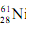has jΠ = 3/2-1 The first excited state at 67.4 keV has jΠ = 1/2-1 and the second excited state at 283.0 keV has List the possible γ -ray transitions between these levels and give their type. Estimate the half-life of the state jΠ = 1/2- using the Weisskopf approximation. How does this compare to the  measured half-life of 23 ps?

2. Quadrupole moments in the shell model. We will calculate an estimate of the quadrupole moment for the special case of a single proton moving in an orbital around a closed shell spherical core. So the only contribution to the quadrupole moment is from this single proton. We will also assume that the proton moves in an orbital with j = l + 1/2 . The space wave function of the proton is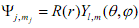where Y is the spherical harmonic, and R is the radial part of the wave function. They are normalized. i.e.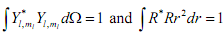(a) Since s mj = m1 + m2, what must m1 and ms be if mj = j?

(b) Show that the quadrupole moment, when m = j, is given by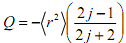To do this start with the quadrupole moment given by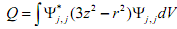.

Write the quadrupole moment operator in terms of Y20 and use the integral,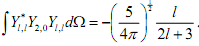(c) Apply this result to the ground state of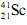, which has jΠ = 7/2-1. Write the configuration for this ground state and confirm that the condition of j = l + ½ holds. Estimate (r2) using r = 1.2 A1/2 . Compare your result to the measured quadrupole moment of -0.156 ± 0.003 b.

(d) Apply this result to the ground state of 917F, which has jΠ = 5/2+. Compare your result to the measured quadrupole moment of 5.8 ± 0.4 fm2.

### Write a Review